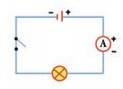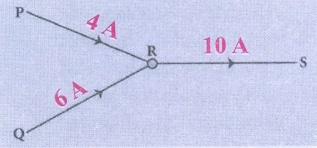Home | | Science 7th Std | Questions Answers

## Chapter: 7th Science : Term 2 Unit 2 : Electricity

7th Science : Term 2 Unit 2 : Electricity : Book Back Questions Answers, Solution

EVALUATION

1. In the circuit diagram below, 10 units of electric charge move past point x every second What is the current in the circuit ___________a. 10 A

a. 1 A

c. 10 V

d. 1 V

2. In the circuit shown, which switches (L,M or N) must be closed to light up the bulb?a. switch L only

b. switch M only

c. Switch M and  Nonly M N

d. either switch L or switches M and N

Answer: d) either switch L or switches M and N

3. Small amounts of electrical current are measured in milliampere  (mA). How many milliampere are there in 0.25 A ?

a. 2.5 mA

b. 25 mA

c. 250 mA

d. 2500 mA

4. In which of the following circuits are the bulb connected in series?II. Fill in the blanks.

1. The direction of conventional current is opposite to electron flow.

2. One unit of coulomb is charge of approximately 6.242 × 1018 protons or electrons.

3. Ammeter is used to measure the electric current.

4. In conducting materials electrons are loosely bounded with atoms.

5. S.I. unit of Electrical conductivity of a conductor is Siemens / meter (S/m).

III. True or False – If False give the correct answer

1. Electron flow is in the same direction to conventional current flow. (False)

Electron flow is in the opposite direction to conventional current flow.

2. The fuse wire does not melts whenever there is overload in the wiring. (False)

The fire wire melts whenever there is overload in the wiring.

3. In a parallel circuit, the electric components are divided into branches.

4. The representation of the electric current is A. (False)

The representation of the electric current is I.

5. The electrical conductivity of the semiconductor is in between a conductor and an insulator. (True)

IV. Match the following

1 .Cell - used to open or close a circuit

2. Switch - safety device used in electric circuit

3. Circuit - A complete path for the flow of an electric current

4. Miniature - Reset by hand, circuit circuit becomes complete once Breaker again

5. Fuse - A device which converts chemical energy into electrical energy

1 .Cell - A device which converts chemical energy into electrical energy

2. Switch - used to open or close a circuit

3. Circuit - A complete path for the flow of an electric current

4. Miniature circuit Breaker - Reset by hand, circuit becomes complete once again

5. Fuse - safety device used in electric circuit

V. Analogy

1. Water : pipe : : Electric current : wire.

2. Copper : conductor : : Wood : non-conductor.

3. Length : metre scale : : Current : Ammeter.

4. milli ampere: micro ampere : : 10-3A : 10-6

VI. Assertion and Reason

1. Assertion (A) : Copper is used to make electric wires.

Reason (R) : Copper has very low electrical resistance.Option:

a. Both A and R are true and R is the correct explanation of A.

b. Both A and R are true but R is NOT the correct explanation of A.

c. A is true but R is false.

d. A is false but R is true.

e. Both A and R are false

Answer: A. Both A and R are true and R is the correct explanation of A.

2. Assertion (A): Insulators do not allow the flow of current through themselves.

Reason (R) : They have no free charge carriers.

a. If both A and R are true and the R is correct explanation of A.

b. If both A and R are true but R is not a correct explanation of A.

c. If A is true and R is false.

d. If both A and R are false.

Answer : B. If both A and R are true but R is not a correct explanation of A.

1. What is the speed of electric current?

280,000,000 meters per second.

2. What is the S.I unit of electrical conductivity?

Siemens/meter (S/m)

3. Name the device used to generate electricity.

Electric cell

4. Define fuse.

The fuse is a wire that melts whenever there is an overload of current in a circuit.

5. Name some devices that run using heat effect of electric current

Electric stove, geyser, room heater, electric iron.

6. Name few insulators.

Rubber, plastics, glass, wood.

7. What is a battery?

A battery is a collection of one or more cells in a circuit.

1. Define an electric current.

An electric current is a flow of electric charge or the amount of charge flowing through a given cross section of a material in unit time.

2. Differentiate parallel and serial circuits.

Parallel Circuit — A circuit in which there are multiple paths for electricity to flow. Each load connected in a separate path receives the full circuit voltage, and the total circuit current is equal to the sum of the individual branch currents.

Series Circuit — A circuit in which there is only one path for electricity to flow. All of the current in the circuit must flow through all of the loads.

3. Define electrical conductivity.

Electrical conductivity or specific conductance is the measure of a material’s ability to conduct an electric current.

1. Explain the construction and working of an Telephone.

In telephones, a changing magnetic effect causes a thin sheet of metal (diaphragm) to vibrate. The diaphragm is made of a metal that can be attracted to magnets.

1. The diaphragm is attached to spring that is fixed to the earpiece.

2. When a current flows through the wires, the soft - iron bar becomes an electromagnet.

3. The diaphragm becomes attracted to the electromagnet.

4. As the person on the other end of the line speaks, his voice causes the current in the circuit to change. This causes the diaphragm in the earpiece to vibrate, producing sound.2. Explain the heating effect of electric current.

When an electric current passes through a wire, the electrical energy is converted to heat.

In heating appliances, the heating element is made of materials with high melting point. An example of such a material is nichrome (an alloy of nickel, iron and chromium).The heating effect of electric current has many practical applications. The electric bulb, geyser, iron box, immersible water heater are based on this effect. These appliances have heating coils of high resistance. Generation of heat due to electric current is known as the heating effect of electricity.

Factors affecting Heating Effect of current

1. Electric Current

2. Resistance

3. Time for which current flows

3. Explain the construction and working of a dry cell.A dry cell is a portable form of a leclanche cell. It consists of zinc vessel which acts as a negative electrode or anode. The vessel contains a moist paste of saw dust saturated with a solution of ammonia chloride and zinc chloride.

The ammonium chloride acts as an electrolyte. (Electrolytes are substances that become ions in solution and acquire the capacity to conduct electricity).

The purpose of zinc chloride is to maintain the moistness of the paste being highly hygroscopic. The carbon rod covered with a brass cap is placed in the middle of vessel. It acts as positive electrode or cathode.

It is surrounded by a closely packed mixture of charcoal and manganese dioxide (MnO2) in a muslin bag. Here MnO2 acts as depolarizer. The zinc vessel is sealed at the top with pitch or shellac. A small hole is provided in it to allow the gases formed by the chemical action to escape. The chemical action inside the cell is the same as in leclanche cell.

X. Higher Order Question

A student made a circuit by using an electric cell, a switch, a torch bulb (fitted in the bulb holder) and copper connecting wires. When he turned on the switch, the torch bulb did not glow at all. The student checked the circuit and found that all the wire connections were tight.

* What could be the possible reason for the torch bulb not glowing even when the circuit appears to be complete?

The filament in the bulb must be fused. The cell may be without electric charge.

XI Picture based Questions

1. Three conductors are joined as shown in the diagram

The current in conductor RS is 10 A. The current in conductor QR is 6 A. What will be the current in conductor PR

a. 4 A

b. 6 A

c. 10 A

d. 16 A2. Draw the circuit diagram for the following series connection3. Study the electric circuit below.  Which  of the following switches should be closed so that only two bulbs will light up

a. S1,S2 and S4 only

b. S1, S3 and S5 only

c. S2, S3 and S4 ony

d. S2, S3 and S5 onlyAnswer : a) S1,S2 and S4 only

4. Study the three electric circuits below. Each of them has a glass rod (G), a steel rod (S), and a wooden rod (W).

In which of the electric circuits would the bulb not light up .

a. A only

b. C only

c. A and B only

d. A , B and CAnswer : c) A and B only

Tags : Electricity | Term 2 Unit 2 | 7th Science , 7th Science : Term 2 Unit 2 : Electricity
Study Material, Lecturing Notes, Assignment, Reference, Wiki description explanation, brief detail
7th Science : Term 2 Unit 2 : Electricity : Questions Answers | Electricity | Term 2 Unit 2 | 7th Science